American Journal of Applied Mathematics
Volume 4, Issue 3, June 2016, Pages: 163-168

Construction of Sc Chordal and Sc Weakly Chordal Graphs

Parvez Ali1, Syed Ajaz Kareem Kirmani2

College of Engineering, Unayzah, Qassim University, Al-Qassim, Kingdom of Saudi Arabia(P. Ali)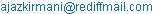(S. A. K. Kirmani)

Parvez Ali, Syed Ajaz Kareem Kirmani. Construction of Sc Chordal and Sc Weakly Chordal Graphs. American Journal of Applied Mathematics. Vol. 4, No. 3, 2016, pp. 163-168. doi: 10.11648/j.ajam.20160403.17

Received: April 13, 2016; Accepted: May 18, 2016; Published: June 4, 2016

Abstract: Study of any graph class includes characterization, recognition, counting the number of graphs i.e. cataloging and construction of graphs. The construction of sc chordal graphs by mean of complementing permutation is one of the known method. In this paper, a new method for the construction of sc chordal graphs is proposed based on a two-pair of graphs. We also presented algorithm for the construction of sc weakly chordal graphs.

Keywords: Self-Complementary Graph, Chordal and Weakly Chordal Graph, Two-Pair, Degree Sequence, P4

Contents

1. Introduction

Let G = (V, E) be an undirected graph with no loops or multiple edges where V(G) and E(G) denote the set of vertices and edges of G respectively. Let S Í V(G) be a set of vertices of G; the subgraph of G induced by S is denoted by G [S]. The neighborhood N(x) of a vertex xÎV(G) is the set of all the vertices of G which are adjacent to x. A path v0, v1, …, vk of a graph is called simple if none of its vertices occurs more than once; it is called a cycle(simple cycle) if v0vkÎE(G). A simple path (cycle) is chordless if vivjÏ E(G) for any two non-consecutive vertices vi, vj in the path (cycle). A chordless path (chordless cycle) on n vertices is denoted by Pn(Cn). Let G be a graph with an induced P4 [a, b, c, d], vertices b, c are called midpoints of the P4 and a, d are called endpoints of the P4. The degree sequence of a graph G is the sequence of the degrees of the n vertices of G arranged in non-increasing order and is denoted by d1 ³ d2 ³³ dn. A pair {x, y} of non-adjacent vertices such that every chordless path from x to y has exactly two edges is known as two-pair. A co pair is a complement of a two pair. Let n be the number of vertices and m be the number of edges in graph G.

A graph is self-complementary (sc) if it is isomorphic to its complement. Every sc graph has 4p or 4p+1 vertex, where p is a positive integer. A graph G is chordal if it has no chordless cycle of length greater than or equal to 4 and it is weakly chordal if both G and its complementhave no chordless cycle of length greater than or equal to 5. A self-complementary graph which is also chordal (respectively, weakly chordal) is called sc chordal graph (resspectively sc weakly chordal graph). For other definitions we refer to .

The construction problem of sc graphs is a fundamental problem in studying sc graphs. The construction of sc graphs by mean of complementing permutation was considered in  and . In  Jin and Wong proposed the decomposition method for the construction of sc graphs. Gibbs  has given algorithms for the construction of sc graphs with 4p and 4p+1 vertices. Sridharan and Balaji  also gave algorithms for the construction of sc chordal graphs with 4p and 4p+1 vertices by modifying the algorithm as discussed in .

There are several techniques discussed in ,  and  for the construction of a graphs having some specific properties. In  X. Huang and Q. Huang gave a method to construct many classes of connected graphs with exactly k- main eigenvalues for any positive integer k. In  Lihuan Mao et. al gave a new method for the construction of graphs by using their generalized spectrum. Recently Honag et. al in  discussed construction of k-critically P5- free graphs.

In this note we study the construction problem for the subclasses of sc perfect graphs, namely sc chordal graphs and sc weakly chordal graphs. Section 2 is devoted to sc chordal graphs where a construction algorithm for sc chordal graphs is propose. In section 3 we study sc weakly chordal graphs and present an algorithm for the construction of sc weakly chordal graphs.

2. Construction of Sc Chordal Graphs

In this section we propose a different approach for the construction of sc chordal graph using the concept of two-pair. Fulkerson and Gross  gave the following classical construction scheme for chordal graphs, which works as follows: "Start with a graph G0 with no vertices and repeatedly add a vertex vj to Gj-1 to create the graph Gj such that vj is not the middle vertex of any P3 of Gj".

This construction scheme for chordal graphs is known as a vertex addition scheme. Now for obtaining the different method for the construction of sc chordal graphs we need the following results.

Theorem 1 . Let G be a sc graph with degree sequence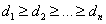. Then G is chordal graph iff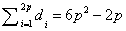for n = 4p &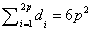for n = 4p + 1.

Theorem 2. . Let G1 be a chordal graph and {x, y} be a pair of non-adjacent vertices of G1. Let G2 be the graph obtained from G1 by adding edge xy; then G2 is chordal iff {x, y} is a two-pair of G1.

Using Theorem-2, We propose the following algorithm-1 for the construction of sc chordal graph with the given degree sequence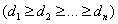. We start with a graph (Gi) having all n isolated vertices and repeatedly add an edge between the non-adjacent vertices (x and y) to create the graph (Gi+1) such that {x, y} is a two-pair in Gi. Algorithm-1 repeats this process until required degree sequence is obtained.

The following algorithm constructs sc chordal graph with the given degree sequence on n vertices.

Algorithm 1. An Algorithm for the construction of sc chordal graph.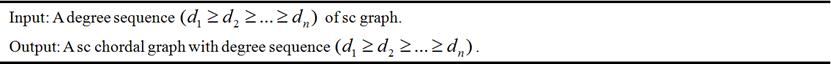Step-1: Start with a graph G1 with n vertices and no edges.

Step-2: Add an edge between two non-adjacent vertices (x and y) of G1, to. obtain graph G2 such that {x, y} is a two-pair in G1.

Step-3: Repeat the process of step-2, until we get a graph with degree sequence.

End.

The following Theorem states that a graph G with n vertices is a sc chordal iff it can be constructed by algorithm-1

Theorem 3.

(i). The constructed graph by algorithm-1 is a sc chordal graph withdegree sequence.

(ii). Every sc chordal graph withdegree sequence on n vertices can be constructed by algorithm-1.

Proof.

(i). The algorithm-1 takes degree sequenceof sc graphs as input and it construct chordal graphs from empty graphs using Theorem-2, which ensures chordality. Also from Theorem-1, no other graph is possible with the same degree sequence.

(ii). Follows from Theorem- 2.

We illustrate algorithm-1 by constructing a sc chordal graph on 12 vertices.

Suppose we have to obtain a sc chordal graph on 12 vertices with degree sequence (10, 10, 8, 8, 6, 6, 5, 5, 3, 3, 1, 1). Algorithm-1 starts with an empty graph G1 on 12 vertices {v1, v2, v3, v4, v5, v6, v7, v8, v9, v10, v11, v12} as shown in figure-1(a). The overall procedure of the construction of sc chordal graph with the given degree sequence (10, 10, 8, 8, 6, 6, 5, 5, 3, 3, 1, 1) is obtained as given below and illustrated in figure-1

(i). The graph G2 as shown in figure-1(b) can be easily obtained, since in G1 there is no edge and in the given degree sequence there is a vertex of degree 10. So we select any vertex (let it be v1) arbitrarily to give it adjacency 10 by adding edges e1, e2, e3, e4, e5, e6, e7, e8, e9, e10 from vertex v1 to v2, v3, v4, v5, v6, v7, v8, v9, v10 and v11 respectively. In this way we get graph G2 as shown in figure-1(b). Obviously there is no violation of step-2 of algorithm-1 by adding these edges in this way.

(ii). In the given degree sequence we have another vertex of degree 10. Let this vertex be v2. Since v2 already got 1 adjacency as can be seen in figure-1(b). So we give it 9 more adjacencies by adding edges e11, e12, e13, e14, e15, e16, e17, e18 and e19 from vertex v2 to v3, v4, v5, v6, v7, v8, v9, v10, v12 respectively. In this way we obtain graph G3 as shown in figure-1(c). From figure-1(b) it is clear that all the non-adjacent pairs i.e. {v2, v3}, {v2, v4}, {v2, v5}, {v2, v6}, {v2, v7}, {v2, v8}, {v2, v9}, {v2, v10} and {v2, v12} are two-pairs in G2. So there is no violation of step-2 of algorithm-1.

(iii). The next lowest degree in the given degree sequence is 8. Let this vertex be v3. Since vertex v3 has already got 2 adjacencies as can be seen in figure-1(c). Therefore we give it 6 more adjacencies by adding the edges e20, e21, e22, e23, e24 and e25 from vertex v3 to v4, v5, v6, v7, v8 and v9 respectively. Doing in this way we obtain graph G4 as shown in figure-1(d). From figure-1 (c) it is clear that all the non-adjacent pairs i.e. {v3, v4}, {v3, v5}, {v3, v6}, {v3, v7}, {v3, v8} and {v3, v9} are two-pairs in the graph G3. So there is again no violation of step-2 of algorithm-1.

(iv). In the given degree sequence there is another vertex of degree 8 (let it be v4). Since vertex v4 has already 3 adjacencies as can be seen in figure-1(d). So we add edges e26, e27, e28, e29 and e30 from vertex v4 to v5, v6, v7, v8 and v10 respectively. In this way we obtain graph G5 as shown in figure-1(e). From figure-1(d) again it is clear that all non-adjacent pairs i.e. {v4, v5}, {v4, v6}, {v4, v7}, {v4, v8} and {v4, v10} are two-pairs in the graph G4.

(v). Now the next lowest degree in the given degree sequence is 6. Let this vertex be v5. From figure-1(e) it is clear that vertex v5 has already adjacency 4, so we have to give only 2 more adjacencies in the form of edges e31 and e32 from vertex v5 to v6 and v7 respectively. Thus we obtain graph G6 in this way as shown in figure-1(f). From figure-1(e) it is clear that non-adjacent pair {v5, v6} and {v6, v7} are two-pairs in the graph G5.

(vi). In the given degree sequence another vertex is of degree 6 (let it be v6). Since vertex v6 already got 5 adjacency, which can be seen in graph G6. So we have to give only 1 adjacency in the form of adding edge e33 from vertex v6 to v8. In this way we obtain graph G7 as shown in figure-1(g). From graph G6 it is clear that the non-adjacent pair {v6, v8} is a two-pair. So adding edge between them is allowed.

As the graph G7 has degree sequence (10, 10, 8, 8, 6, 6, 5, 5, 3, 3, 1, 1) which is the same degree sequence given in the input of algorithm-1. Thus algorithm stops here. Since there is no violation of step-2 at any stage of construction, therefore the constructed graph G7 is sc chordal on 12 vertices with degree sequence (10, 10, 8, 8, 6, 6, 5, 5, 3, 3, 1, 1).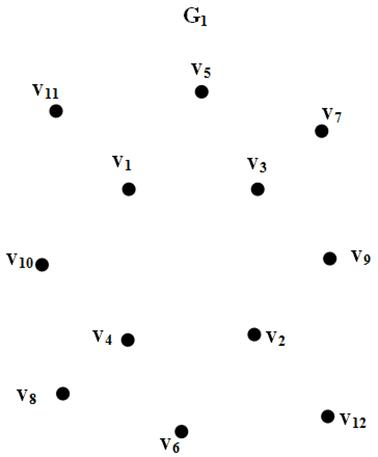(a)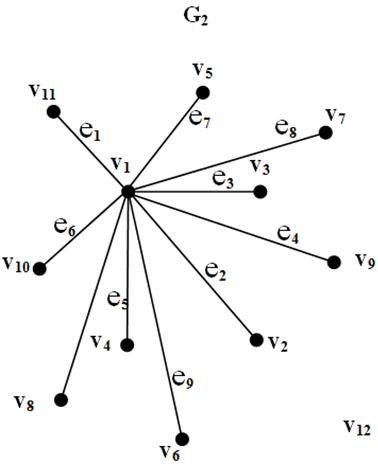(b)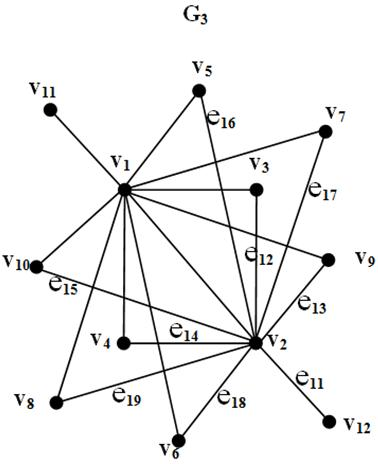(c)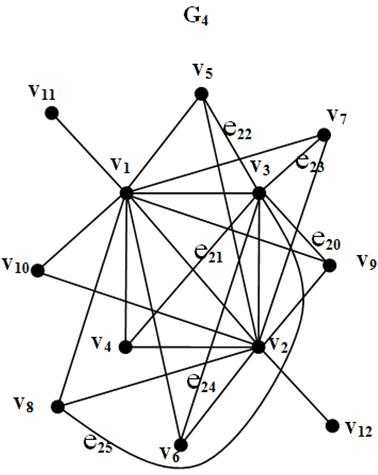(d)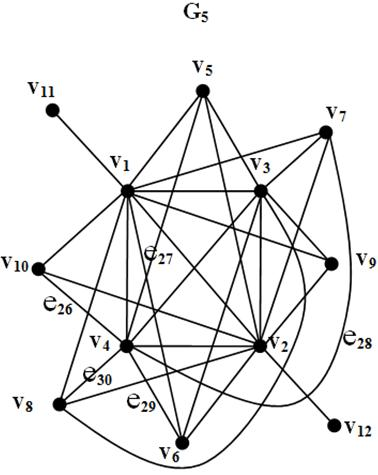(e)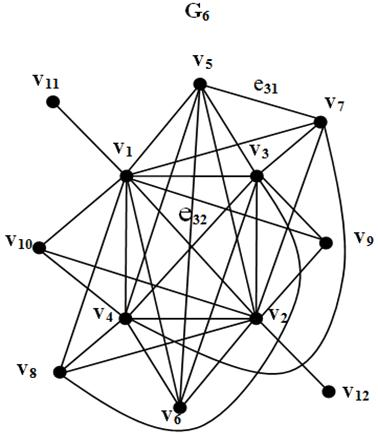(f)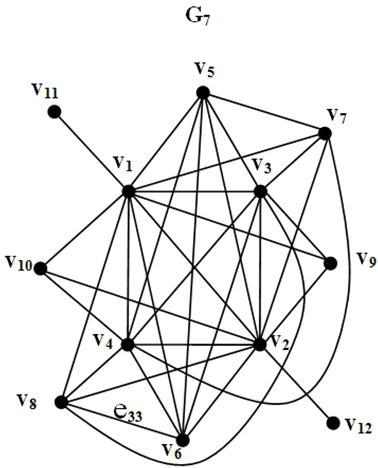(g)

Figure 1. Construction of sc chordal graph with the given degree sequence (10,10,8,8,6,6,5,5,3,3,1,1)

3. Construction of Sc Weakly Chordal Graphs

In  Hayward had established a structural relationship between chordal graphs and weakly chordal graphs by defining a construction scheme on weakly chordal graphs. He noted that the class of chordal graphs can be generated by repeatedly adding a vertex which is not the middle vertex of P3 and showed that weakly chordal graphs can likewise be generated by starting with a set of vertices and no edges and repeatedly adding an edge which is not the middle edge of P4.

The following Theorem states Hayward  result in more appropriate way.

Theorem 4. A graph is weakly chordal graph iff it can be generated in the following manner

(ii). Repeatedly add an edge ej to Gj-1 to create the Gj such that ej is not the middle edge of any P4 of Gj.

We give an algorithm using above result for the construction of a sc weakly chordal graph with a given degree sequenceof sc graph with n vertices. The algorithm-2 work as follows: it starts with a graph Gi with no edges on n vertices. Then step-2 repeatedly adds an edge ei between any two vertices to obtain the graph Gi+1 such that the added edge is not the middle edge of any P4. Repeating this process if the algorithm obtains the degree sequence as given in input of the algorithm, then it stops and produces a sc weakly chordal graph.

The following algorithm constructs sc weakly chordal graph with the given degree sequence on n vertices.

Algorithm 2. An Algorithm for the construction of sc weakly chordal graph.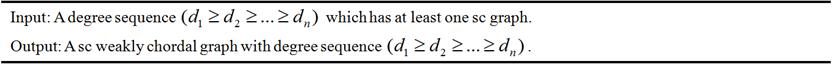Step-1: Start with a graph G1 on n vertices and no edges.

Step-2: Add an edge ei between any two vertices such that added edge ei is not middle edge of any P4 of Gi.

Step-3: Repeat the process of step-2, until to get a graph with degree sequence.

End.

We illustrate algorithm-2 by constructing a sc weakly chordal graph on 9 vertices.

Suppose we have a degree sequence (6, 6, 4, 4, 4, 4, 4, 2, 2) and we want to obtain a sc weakly chordal graph of this degree sequence.

Algorithm-2. starts with 9 vertices as {v1, v2, v3, v4, v5, v6, v7, v8, v9} and no edges as shown by graph G1 in figure-2(a). We can add edges on this graph G1 one by one such that added edge is not the middle edge of any P4 as the rule of step-2 of algorithm-2. The overall procedure of the construction of sc weakly chordal graph with the given degree sequence (6, 6, 4, 4, 4, 4, 4, 2, 2) is obtained as given below and illustrated in figure-2.

(i). The graph G2 as shown in figure-2(b) can be easily obtained by adding edges e1, e2, e3, e4, e5 and e6 between the vertices {v1, v2}, {v1, v3}, {v1, v4}, {v1, v5}, {v1, v6} and {v1, v8} respectively. From the figure-2(b) it is clear that none of the added edges are the middle edges of any P4.

(ii). In the graph G3 as shown in figure-2(c), added edges are e7, e8, e9, e10 and e11. Edge e7, e8 and e9 are not the middle edges of P4 since they are part of the triangle among the vertices {v1, v2, v3}, {v1, v2, v4} and {v1, v2, v6} respectively. Edges e10 and e11 are obviously not the middle edges of any P4 since they have vertex v7 and v9 of degree 1 respectively.

(iii). In the graph G4 as shown in figure-2(d), the added edges are e12 and e13. Edges e12 and e13 are not the middle edges of P4 since they are part of triangle among the vertices {v1, v3, v5} and {v2, v3, v9} respectively.

(iv). The next graph G5 as shown in figure-2(e) has added edges as e14 and e15. Both edges are the part of triangle among the vertices {v1, v4, v5} and {v2, v4, v7} respectively.

(v). In the graph G6 as shown in figure-2(f) the only added edge is e16. Edge e16 is not the middle edge of any P4 since it is part of the chordless cycle of length 4 between the vertices v2, v3, v5, and v7.

(vi). The next graph G7 as shown in figure-2(g) has added edges as e17 and e18. Both edges are the part of triangle among the vertices {v2, v6, v7} and {v1, v6, v8} respectively.

The algorithm-2 stops here, since graph G7 has degree sequence (6, 6, 4, 4, 4, 4, 4, 2, 2) which is the same degree sequence as given in input of the algorithm. Since there is no violation at any stage of the algorithm, so the constructed graph G7 is sc weakly chordal graph with the degree sequence (6, 6, 4, 4, 4, 4, 4, 2, 2) on 9 vertices.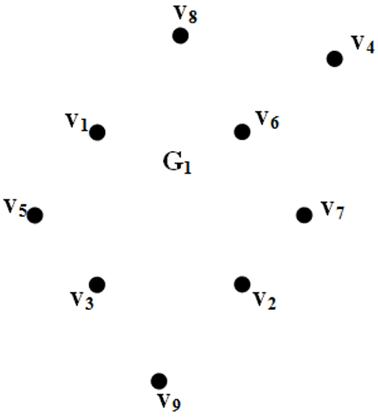(a)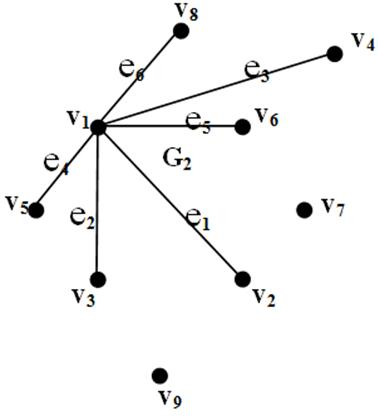(b)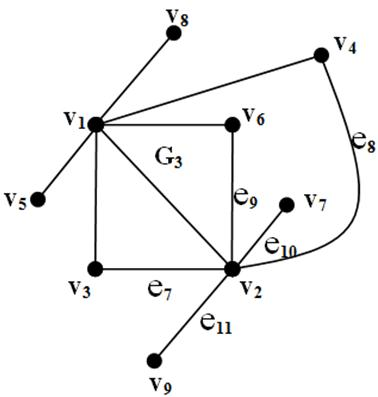(c)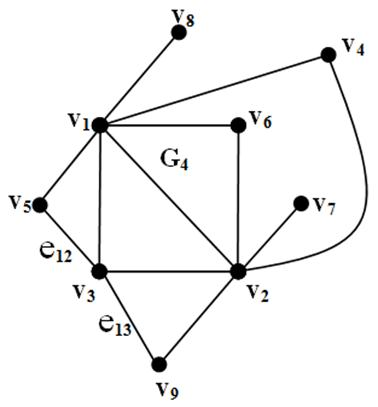(d)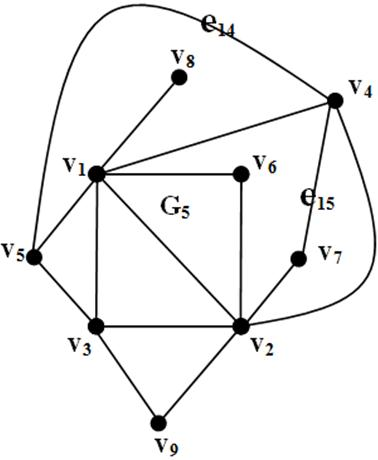(e)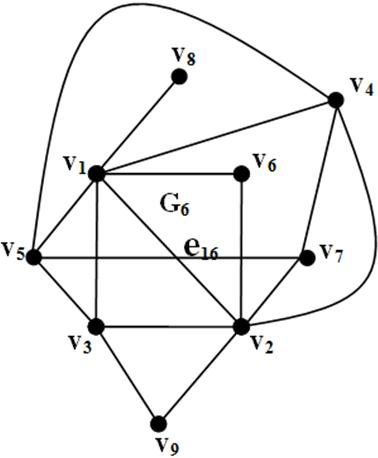(f)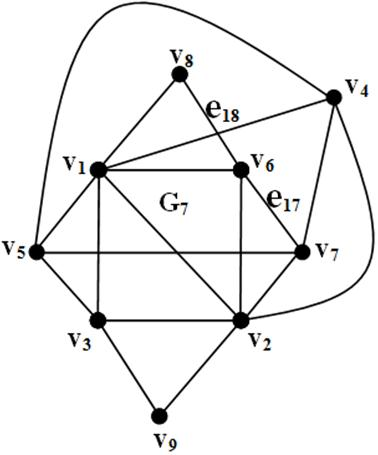(g)

Figure 2. Construction of sc weakly chordal graph with the given degree sequence (6,6,4,4,4,4,4,2,2).

4. Conclusion

In  the given algorithms construct the same graph many times. So in order to, compile a catalogue of sc chordal graphs with 4p+1 vertices, every pair of the constructed graphs should be tested whether the graphs are isomorphic or not after constructing all sc chordal graphs with 4p+1 vertices from the given algorithms.

The algorithm-1 given in section 2 takes input a degree sequence of sc graph and throughout the process the chordality of graphs is not violated. Also from theorem-1 no other graph is possible with the same degree sequence. So it is eay to construct all the sc chordal graph by the algorithm-1 as compare to algorithm given in .

References

1. A. Berry, A. Sigayret and C. Sinoquet, Maximal sub-triangulation as pre-processing phylogentic data, Soft computing 10 (2006) 461-468.
2. C. T. Hoang, Brain Moore, D. Roecoskie, J Sawada, M. Vatshelle, Construction of k- critical P5-free graphs, Discrete Applied Mathematics, 182 (2015), 91-98.
3. D. R. Fulkerson and O. A. Gross, Incidence matrices and interval graphs, Pacafic J. Math., 15 (1965) 835-855.
4. G. Ringel, Selbstkomplementare Graphen, Arch Math. (Basel), 14 (1963) 354-358.
5. H. Sachs, Uber selbstkomplementare Graphen, Publ. Math. Debreen, 9 (1962) 270-288.
6. J. Yellen and J Gross, Graph Theory and its Applications, CRC Press (USA), 1999.
7. J. Xu and C. K. Wong, Self-complementary graphs and Ramsey numbers Part-1: the decomposition and the construction of self-complementary graphs, Discrete mathematics, 223 (2000) 309-326.
8. Lihuan Mao, Fenjin Liu, Wei Wang, A new method for the constructing graphs determined by their generalized spectrum, Linear Algebra and its Applications, 477 (2015) 112-127.
9. M. R. Sridharan and K. Balaji, Characterisation of self-complementary chordal graphs, Discrete Mathematics, 188 (1998) 279-283.
10. M. R. Sridharan and K. Balaji, On construction of self-complementary chordal graphs, Journal of Combinatorics Information and system sciences, 24 (1999) 39-45.
11. R. A. Gibbs, Self-Complementary Graphs, J. Comb. Theory Series B, 16 (1974) 106-123.
12. R. B. Hayward, Generating weakly triangulated graphs, J. Graph Theory, 21 (1996) 67-70.
13. Xueyi Huang and Qiongxiang Huang Construction of graphs with exactly k main eigenvalues, Linear Algebra and its Applications, 486 (2015), 204-218

 Contents 1. 2. 3. 4.
Article ToolsAbstractPDF(1383K)